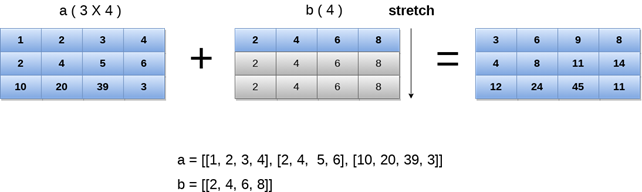In Mathematical operations, we may need to consider the arrays of different shapes. NumPy can perform such operations where the array of different shapes are involved.

For example, if we consider the matrix multiplication operation, if the shape of the two matrices is the same then this operation will be easily performed. However, we may also need to operate if the shape is not similar.

Consider the following example to multiply two arrays.

### Example

``````import numpy as np
a = np.array([1,2,3,4,5,6,7])
b = np.array([2,4,6,8,10,12,14])
c = a*b;
print(c)
``````

Output:

`

[ 2 8 18 32 50 72 98]

`
However, in the above example, if we consider arrays of different shapes, we will get the errors as shown below.

Example

``````import numpy as np
a = np.array([1,2,3,4,5,6,7])
b = np.array([2,4,6,8,10,12,14,19])
c = a*b;
print(c)
``````

Output:

`

ValueError: operands could not be broadcast together with shapes (7,) (8,)

`

In the above example, we can see that the shapes of the two arrays are not similar and therefore they cannot be multiplied together. NumPy can perform such operation by using the concept of broadcasting.

In broadcasting, the smaller array is broadcast to the larger array to make their shapes compatible with each other.

Broadcasting is possible if the following cases are satisfied.

1. The smaller dimension array can be appended with ‘1’ in its shape.
2. Size of each output dimension is the maximum of the input sizes in the dimension.
3. An input can be used in the calculation if its size in a particular dimension matches the output size or its value is exactly 1.
4. If the input size is 1, then the first data entry is used for the calculation along the dimension.

Broadcasting can be applied to the arrays if the following rules are satisfied.

1. All the input arrays have the same shape.
2. Arrays have the same number of dimensions, and the length of each dimension is either a common length or 1.
3. Array with the fewer dimension can be appended with ‘1’ in its shape.

Let’s see an example of broadcasting.

### Example

``````import numpy as np
a = np.array([[1,2,3,4],[2,4,5,6],[10,20,39,3]])
b = np.array([2,4,6,8])
print("\nprinting array a..")
print(a)
print("\nprinting array b..")
print(b)
print("\nAdding arrays a and b ..")
c = a + b;
print(c)
``````

Output:

``````printing array a..
[[ 1  2  3  4]
[ 2  4  5  6]
[10 20 39  3]]

printing array b..
[2 4 6 8]

Adding arrays a and b ..
[[ 3  6  9 12]
[ 4  8 11 14]
[12 24 45 11]]
``````